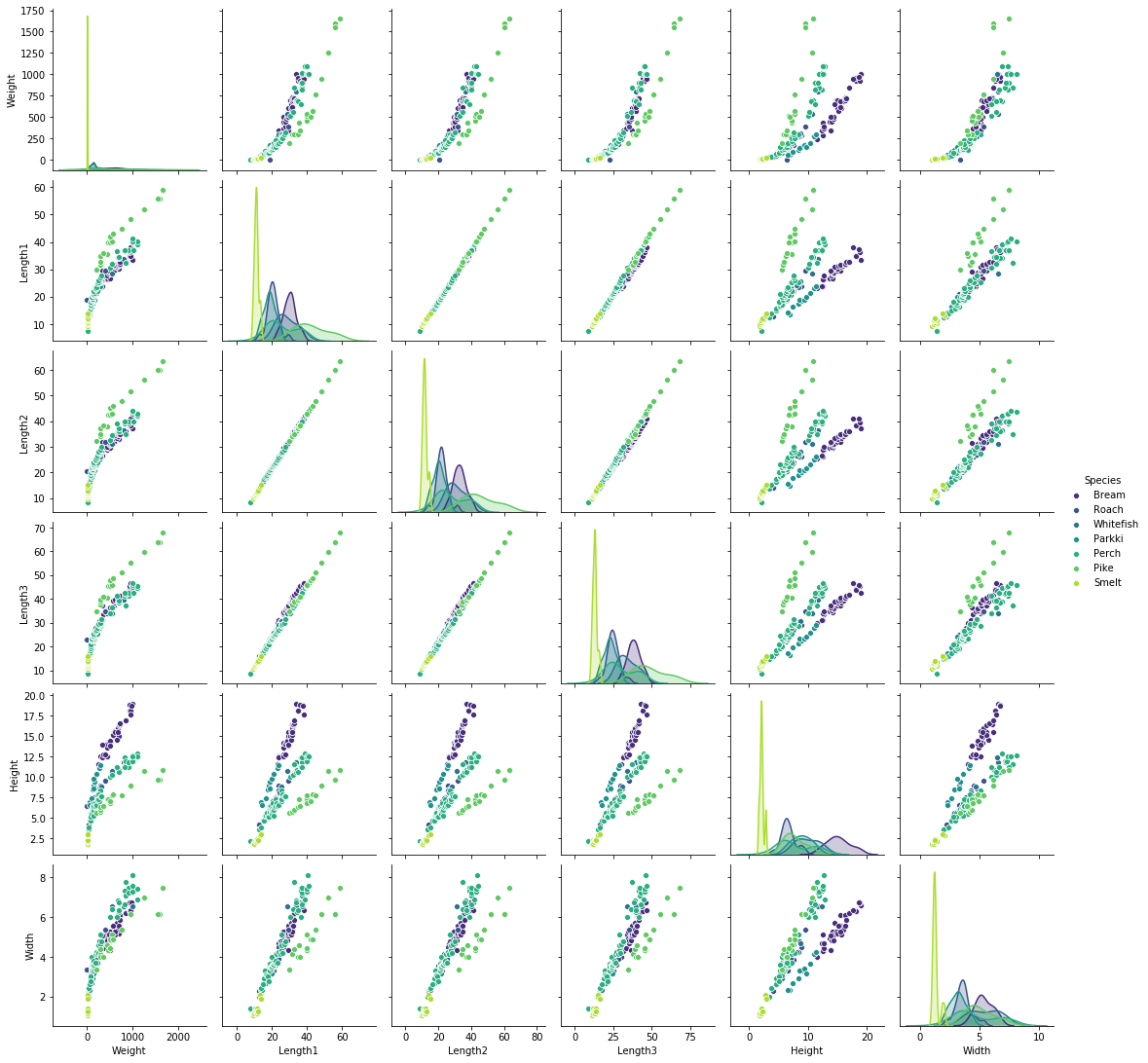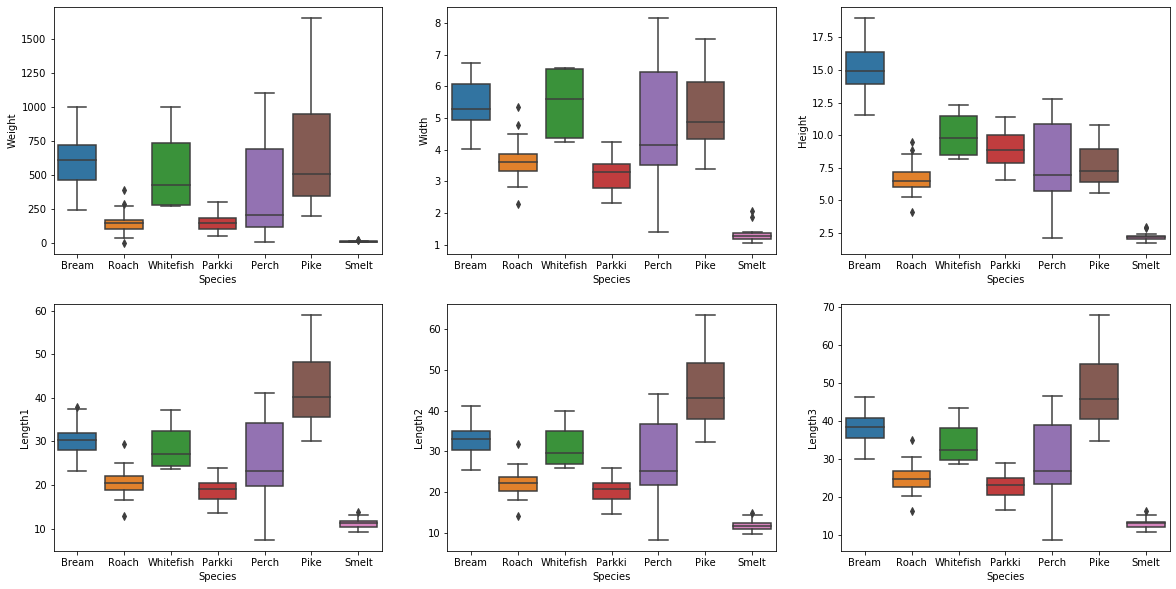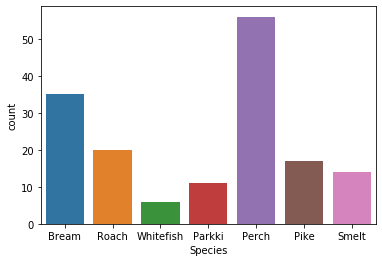# Machine Learning 101: K-Nearest Neighbors in Python (Classification)K-Nearest Neighbors is a double-edged algorithm that can be used for both Classification and Regression problems. While its intuition is quite simple, it can yield impressive results, but there are a few drawbacks, hence double-edged.

## Classification vs Regression

Machine Learning essentially deals with two kinds of problems:

• Classification: predicting a class, for example whether a user is male or female (the two classes) given their history of purchased items.
• Regression: predicting a value, for example the price (the value) of a used car given the model, the age, the kilometers on the odometer.

It is important to remember that Machine Learning is no magic, ML algorithms are still algorithms: multiple inputs, one output. The most important difference between a traditional algorithm and an ML one is the “experience” the ML algorithm gains during the training phase.

In Classification problems the algorithm tries to predict the class the entry will fall into, it may be two classes (such as the example above, male versus female) or more than two classes. The former is often called Binary Classification the latter is referred to as Multiclass Classification.

In Regression there is no class to predict, instead there is a scale and the algorithm tries to predict the value on that scale. In the example above the price is the sought value.

## K-Nearest Neighbors (Classification) in Python

The intuition behind K-Nearest Neighbors is quite simple: if the observation is close to others observations (with a minimum of K) it must be of the same kind.

Imagine a square where three stalls are performing different magic tricks, there are illusionists, fire-dancers and tamers.

Now imagine a worker for one of the three stalls that has just come and he’s late, he goes in the center of the square and finds its stall then takes a step.

You can use KNN to determine which stall the worker will go to by calculating the distance between the worker and all the other workers. If there are enough colleagues (let’s say 2) within his range and they’re the closest it will mean he probably has seen its colleagues and he belongs there. Now this seems probably obvious since he has taken a step towards the stall, but the real difference here is the distance between the worker and each other worker in the square.

By taking a step towards his colleagues the worker has decreased the distance between himself and its colleagues and has increased the distance between himself and the other groups.

You might also have noticed an important thing about KNN, k is chosen by you and the right number for K actually depends on the data.

The distance is therefore essential to determine whether a new observation will befall a certain category or not. Historically the Euclidean Distance has been used while nowadays the Minkowski Distance is used (it is a generalization of both Euclidean and Manhattan distances). Here’s the formula to calculate the Euclidean Distance between p and q in an n-dimensional space:

$$d\left(p,q\right) = \sqrt{\sum _{i=1}^{n} \left(q_{i}-p_{i}\right)^2}$$

## K-Nearest Neighbors using fish (classification problem)

The following notebook uses the Fish market dataset available here, it is free and released under the GPL2 license. This dataset includes a number of species of fish and for each fish some measurements such as weight and height.

### (Basic) Explanatory Data Analysis

Every good ML algorithm should start with an in-depth Explanatory Data Analysis (EDA). In the EDA you should always try to explore the data as much as possible, through exploration it is possible to infer basic features of the data, from those basic inferences you can start developing a basic intuition. From there you can start formulating hypotheses and implement the algorithm you see fit.

As the purpose of this notebook is to illustrate K-Nearest Neighbors applied to a Classification Problem, the performed EDA will outline just the basic features of the dataset.

Firstly, let's import basic utilities:

In :
%matplotlib inline
import pandas as pd
import numpy as np
import matplotlib.pyplot as plt
import seaborn as sns

np.random.seed(101) # This is needed so that if you run this notebook again you will get the same results


Let's now read the csv file (keep in mind it should be in the same folder as the notebook!):

In :
df = pd.read_csv('Fish.csv')


Let's take a look at the first rows of the dataset. It is important to do this in order to get a basic understanding.

In :
df.head()

Out:
Species Weight Length1 Length2 Length3 Height Width
0 Bream 242.0 23.2 25.4 30.0 11.5200 4.0200
1 Bream 290.0 24.0 26.3 31.2 12.4800 4.3056
2 Bream 340.0 23.9 26.5 31.1 12.3778 4.6961
3 Bream 363.0 26.3 29.0 33.5 12.7300 4.4555
4 Bream 430.0 26.5 29.0 34.0 12.4440 5.1340

Now let's take a closer look to the dataset to get important statistical indicators such as the mean and standard deviation

In :
df.describe()

Out:
Weight Length1 Length2 Length3 Height Width
count 159.000000 159.000000 159.000000 159.000000 159.000000 159.000000
mean 398.326415 26.247170 28.415723 31.227044 8.970994 4.417486
std 357.978317 9.996441 10.716328 11.610246 4.286208 1.685804
min 0.000000 7.500000 8.400000 8.800000 1.728400 1.047600
25% 120.000000 19.050000 21.000000 23.150000 5.944800 3.385650
50% 273.000000 25.200000 27.300000 29.400000 7.786000 4.248500
75% 650.000000 32.700000 35.500000 39.650000 12.365900 5.584500
max 1650.000000 59.000000 63.400000 68.000000 18.957000 8.142000

Let's now plot each numerical feature against each other, in order to get a clear distinction use a palette with high contrast (Viridis) and use the species as color.

In :
sns.pairplot(df, hue='Species', palette='viridis')

Out:
<seaborn.axisgrid.PairGrid at 0x1db797e48c8>As you can see there is quite a strong pattern between: Length1 and Length2, Length2 and Length3, Length3 and Length1.

In the next step we will try to predict whether the fish is a Perch or a Bream, so let's get a better look at each feature for each species.

In :
fig, axes = plt.subplots(ncols=3, nrows=2, figsize=(20, 10), sharey=False)
sns.boxplot(data=df, y='Weight', x='Species', ax=axes)
sns.boxplot(data=df, y='Width', x='Species', ax=axes)
sns.boxplot(data=df, y='Height', x='Species', ax=axes)
sns.boxplot(data=df, y='Length1', x='Species', ax=axes)
sns.boxplot(data=df, y='Length2', x='Species', ax=axes)
sns.boxplot(data=df, y='Length3', x='Species', ax=axes)

Out:
<matplotlib.axes._subplots.AxesSubplot at 0x1db7b1b3948>As you can see Length1, Length2 and Length3 follow essentially the same pattern across each species (hence they contain almost the same information). On the other hand each species has a set of Width, Height and Weight.

As the dataset is quite small it may be worth knowing how many observations there are for each fish species:

In :
sns.countplot(data=df, x='Species')

Out:
<matplotlib.axes._subplots.AxesSubplot at 0x1db7c008248>### K-Nearest Neighbours (binary classification): Let's predict whether the fish is a Perch or a Bream

In this step a KNN model to predict the fish species will be created. In order to make it simpler for the model to make predictions we will restrict the domain of species to Perch and Bream species. The model will be able to tell a Perch apart from a Bream, but any other fish it will have no clue.

The following lines allow us to create a X variable containing all the features except "Species", and a y variable containing just the species. Both variables will only include observations about Perch and Bream species.

In :
X = df[(df['Species'] == 'Perch') | (df['Species'] == 'Bream')].drop('Species', axis=1)
y = df[(df['Species'] == 'Perch') | (df['Species'] == 'Bream')]['Species'].replace(['Bream', 'Perch'], [0, 1])


Split the dataset in two parts: train and test. This is needed to calculate the accuracy (and many other metrics) of the model. We will use the train part during the training, and the test part during the evaluation. The model will not see the test part during its training.

In :
from sklearn.model_selection import train_test_split
X_train, X_test, y_train, y_test = train_test_split(X, y, test_size = 0.33, random_state=101)


Without delving too much into mathematics, using KNN involves using a metric to calculate the distance between a point and its neighbours, this metric is usually the Euclidean Distance. In order for the features to be equally important, each feature should be standardized. You can standardize any dataset by doing:

$$\mathcal{z}=\frac{x-\mu}{\sigma}$$

Where $\mu$ is the mean average and $\sigma$ is the standard deviation of each particular column. You can work the math for each column and assign them using pandas features, or you can leverage sklearn StandardScaler. The StandardScaler will first need to be fitted (calculate $\mu$ and $\sigma$). Then the scaler will be used to scale X_train and X_test. You don't need to scale the y variable (it also only has boolean values in it (0, 1). Another thing you don't want to do is fit the scaler on train and test data, by doing this you will throw off the parameters and "leak" information (you will inherently tell bits of how the X_test looks like). Once the scaler has been fitted to the data, the data can be transformed using the formula above.

In :
from sklearn.preprocessing import StandardScaler

scaler = StandardScaler()
scaler = scaler.fit(X_train)
X_train = scaler.transform(X_train)
X_test = scaler.transform(X_test)


Import the model and instantiate it:

In :
from sklearn.neighbors import KNeighborsClassifier

knc = KNeighborsClassifier(2, p=2)


Now let's train the model:

In :
knc.fit(X_train, y_train)

Out:
KNeighborsClassifier(algorithm='auto', leaf_size=30, metric='minkowski',
metric_params=None, n_jobs=None, n_neighbors=2, p=2,
weights='uniform')

In order to review our model we will use two handy functions: the classification report (which sums up useful statistics, and the confusion matrix.

In :
from sklearn.metrics import classification_report, confusion_matrix, f1_score

In :
print(classification_report(y_test, knc.predict(X_test)))

              precision    recall  f1-score   support

0       1.00      1.00      1.00        14
1       1.00      1.00      1.00        17

accuracy                           1.00        31
macro avg       1.00      1.00      1.00        31
weighted avg       1.00      1.00      1.00        31



While discussing about the matrics deserves a whole article by itself, it is easy to observe the model is a perfect fit, achieving 100% precision and recall. The "support" column tells you how many observation support the class (0, 1). Let's now take a look at the confusion matrix:

In :
print(confusion_matrix(y_test, knc.predict(X_test)))

[[14  0]
[ 0 17]]


While the classification report allows for a quick review of the model performance, the confusion matrix takes a bit more to be understood.

• The upper left number represents true positives (they are 1, classified as 1)
• The upper right number represents false positives (they are 0, classified as 1)
• The lower left number represents false negatives (they are 1, classified as 0)
• The lower right number represents true negatives (they are 0, classified as 0)

#### Conclusion: It's prefect!

Although the model can only predict whether the fish is a Perch or a Bream, it seems to be working perfectly, but is it really? First of all as you can saw during the EDA, Breams and Perches are essentially different fishes. You should also keep in mind the input of the model (weight, length1, length2, length3, width and height) is essentially shared among ALL fishes in the world. Imagine you find a fish whose features are essentially the same of a Bream but it is not a Bream. Well, the model would classify it as a Bream, making an error. Since the model trained on Bream and Perch species only (albeit with a very small dataset), you should be sure you're trying to classify one of the two.

### K-Nearest Neighbors (multiclass problem): Let's predict the species

This problem closely resembles the last one, yet we're now trying to predict the species. There are seven species in this dataset and there aren't many observations for some of them.

In :
X = df.drop(['Species'], axis=1)
y = df['Species']

In :
from sklearn.model_selection import train_test_split
X_train, X_test, y_train, y_test = train_test_split(X, y, test_size = 0.33, random_state=101)

In :
from sklearn.preprocessing import StandardScaler

scaler = StandardScaler()
scaler = scaler.fit(X_train)
X_train = scaler.transform(X_train)
X_test = scaler.transform(X_test)

In :
from sklearn.neighbors import KNeighborsClassifier

knc = KNeighborsClassifier(2, p=2)

In :
knc.fit(X_train, y_train)

Out:
KNeighborsClassifier(algorithm='auto', leaf_size=30, metric='minkowski',
metric_params=None, n_jobs=None, n_neighbors=2, p=2,
weights='uniform')

Let's now evaluate the model:

In :
from sklearn.metrics import classification_report

In :
print(classification_report(y_test, knc.predict(X_test), zero_division=0))

              precision    recall  f1-score   support

Bream       0.90      1.00      0.95         9
Parkki       1.00      0.33      0.50         6
Perch       0.79      0.95      0.86        20
Pike       1.00      1.00      1.00         5
Roach       0.38      0.43      0.40         7
Smelt       1.00      0.80      0.89         5
Whitefish       0.00      0.00      0.00         1

accuracy                           0.79        53
macro avg       0.72      0.64      0.66        53
weighted avg       0.80      0.79      0.77        53



Where's the confusion matrix? If you print the confusion matrix for a multiclass problem you will see it is actually quite difficult to interpret its results, stick to the classification report for easier-to-understand values. For the curious ones:

In :
print(confusion_matrix(y_test, knc.predict(X_test)))

[[ 9  0  0  0  0  0  0]
[ 1  2  0  0  3  0  0]
[ 0  0 19  0  1  0  0]
[ 0  0  0  5  0  0  0]
[ 0  0  4  0  3  0  0]
[ 0  0  1  0  0  4  0]
[ 0  0  0  0  1  0  0]]


### Conclusion: Not so accurate now?

As the previous model achieved 100% accuracy and recall, the second one achieved an overall accuracy of 0.79. As you can see as the number of species grow and the features overlap, the model struggles to distinguish between fish species with some species such as Roach and Whitefish being mispredicted all of the times. Also Perches and Breams that were previously classified perfectly are now not as certain as before. The size of the dataset, the presence of outliers, the weaknesses of the model all show up together in the last report.

## Conclusion

KNN is a great algorithm that can yield impressive results but its complexity quickly grows as the number of dimensions and observations grows. It is by many considered the go-to ML algorithm, but it should not be used blindly. In the notebook shown above the features show a linear pattern and that should be an indicator that KNN will not perform well. Since there is a strong linear correlation between many features, other techniques such as Linear Regression or Logistic Regression should come to mind first.

Image courtesy of mark | marksei

The following two tabs change content below.#### mark

The IT guy with a slight look of boredom in his eyes. Freelancer. Current interests: Kubernetes, Tensorflow, shiny new things.#### You may also like...

This site uses Akismet to reduce spam. Learn how your comment data is processed.